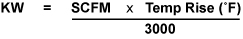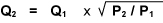# Air Heater Power and Flow Graphs

## Power Required To Heat Air

An approximation of air heating power requirements can be calculated with the following formula:## Flow Meter Correction Curve

All Performance Curves for CONVECTRONICS Air Heaters refer to “Actual” Air Flow Rates (SCFM). The pressure at the Flow Meter outlet should be measured and the “Observed” Flow should be adjusted per correction curves to obtain “Actual” Flow. The Actual Flow can also be calculated by using the following formula:An approximation of air heating power requirements can be calculated with the following formula:Q1 = Observed Flow
Q2 = Actual Flow Corrected for Pressure
P1 = Atmospheric Pressure, 14.7 PSI
P2 = Actual Pressure, 14.7 PSI + Pressure at Flow Meter Outlet

•